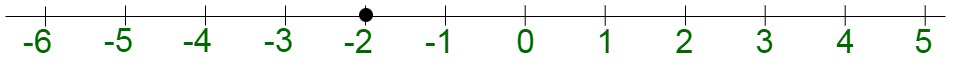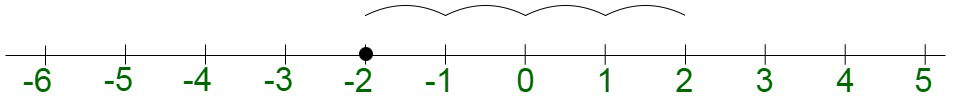# What is the rule for subtracting negative numbers?

• Last Updated : 02 Dec, 2021

Algebra is the branch of mathematics dealing with arithmetic operations and its associated symbols. The symbols are termed as variables that may take different values when subjected to different constraints. The variables are mostly denoted such as x, y, z, p, or q, which can be manipulated through different arithmetic operations of addition, subtraction, multiplication, and division, in order to compute the values.

### Negative Numbers

Negative Numbers are denoted by integers prepended by a minus sign. For instance, -4, -2 are negative numbers. Negative numbers lie on the left side of the number line, they are separated with the positive numbers by 0. It can be said that negative numbers are the complement of positive numbers. The negative numbers can be easily added or subtracted by using both the negative operands. Let’s learn how to specifically subtract negative numbers with proper cases,

### What is the rule for subtracting negative numbers?

Solution:

Rule 1: Subtracting a negative number from a negative number (-) a minus sign followed by a negative sign, turns the two signs into a plus sign.

Subtraction of a negative number from another negative number is simply an addition of negative and positive numbers. This is because, according to the known rule, – (-4) becomes +4. The resultant operation becomes positive in nature. The final operation may be positive or negative in nature. However, the magnitude of the final output is greater than both the operands, in case none of the operands is 0. In the case of subtracting negative numbers, the following scenarios may arise where we are subtracting the second operand from the first operand:

• Second operand > First operand
In case the magnitude of the second operand is greater than the first operand, the final output has a positive sign associated with it. For example, we have, -2 – (-4). This equation is equivalent to -2 + 4, which boils down to the addition of 4 to -2. On the number line, it starts at -2.Then we move forward with 4 units: +4.The answer is -2 – (-4) = 2.

• Second operand < First operand
In case the magnitude of the second operand is greater than the first operand, the final output has a negative sign associated with it. For example, we have, -4 – (-2). This equation is equivalent to -4 + 2, which boils down to the addition of 2 to -4. On the number line, it starts at -4. On addition of 2, the result becomes -2.
• Second operand = First operand
In case the magnitude of second operand is equal to the first operand, the final output is 0. For example, we have, -2 – (-2). This equation is equivalent to -2 + 2, which boils down to the addition of 2 to -2 and produces 0.

### Sample Problems

Question 1: Evaluate -4 – (-10) – 2 – (-25).

Solution:

-4 – (-10) – 2 – (-25)

• First open the brackets./ul>

= -4 + 10 – 2 + 25

• Add the positive and negative integers separately.

= -4 – 2 + 10 + 25

= -6 + 35

= 29

Question 2: Find the solution for: (2 × 2) – (3 × 3) – (4 × 4)

Solution:

(2 × 2) – (3 × 3) – (4 × 4)

• First solve the brackets.

= (4) – (9) – (16)

• Now open the brackets.

= 4 – 9 – 16

• Add the positive and negative integers separately.

>

= 4 – 25

= -21

Question 3: Subtract (2x + 3y)2 from (4x – 5y)2.

Solution:

(4x – 5y)2 – (2x + 3y)2

• Solve the brackets.

Using algebraic identity,

(x + y)2 = x2 + y2 + 2xy

= (16x2 + 25y2 – 40xy) – (4x2 + 9y2 + 12xy)

• Now open the brackets

= 16x2 + 25y2 – 40xy – 4x2 – 9y2 – 12xy

• Now add or subtract the like terms

= 16x2 – 4x2 + 25y2 – 9y2 – 40xy – 12xy

= 12x2 + 16y2 – 52xy

Question 4: Subtract (6x – 8y)2 from 2x2 &#

; 4y2 – 12xy

Solution:

2x2 – 4y2 – 12xy – (6x – 8y)2

• Solve the bracket.

Using algebraic identity,

(x + y)2 = x2 + y2 + 2xy

= 2x2 – 4y2 – 12xy – (36x2 + 64y2 – 96xy)

• Open the bracket.

= 2x2 – 4y2 – 12xy – 36x2 – 64y2 + 96xy

• Add or subtract like terms.

= 2x2 – 36x2 – 4y2 – 64y2 – 12xy + 96xy

= -34x2 – 68y2 + 84xy

My Personal Notes arrow_drop_up